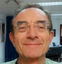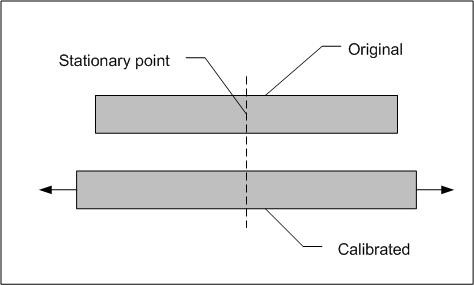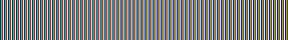Search
•Yitzhak Weissman

# Have a 60.02 pitch lens? This is how to make the best of it!

Having a lens with a very small deviation from a nominal pitch value, like 60.02/inch, could be a real jackpot. However, even such a small deviation can deny you the prize if the interlaced image is not calibrated properly.

Calibration is an inevitable step in lenticular print processing. Its role is to assure that the printed grid matches the lenticular grid. The calibration process can be visualized by imagining that the interlaced image is printed on an elastic material. To calibrate it, this elastic picture is stretched (or contracted) until the required matching is achieved.

In reality, the calibration is carried out by numerical resizing pf the interlaced image. However, in contrast to physical stretching of an elastic picture, numerical resizing causes loss of resolution, due to an action called "resampling".

The amount of resizing is the primary characteristic of the calibration. However, contrary to the popular impression, it is not the only one. In resizing, one can choose a stationary point on the image, which is a point that remains intact during the process. This characteristic of the calibration process is generally overlooked.

Common resizing programs do not allow the user to choose the stationary point. In all cases known to the author, these programs choose one of the image edges as stationary points. An example of stretching with the left edge stationary is illustrated in Figure 1.Figure 1: Stretching with a stationary left edge

Figure 2 illustrates central stretching, in which the central point remains stationary.Figure 2: Stretching with a stationary center

The reason why the location of the stationary point is significant is that at this point there is no loss of resolution. In 3D lenticular pictures, higher resolution can be used to display more depth. In most cases, the artist will prefer to have the highest resolution at the picture center, rather than somewhere else, thus preferring centered calibration.

The resolution remains high not only at the stationary point itself but also in its neighborhood. The width of this sharp zone depends on the magnitude of the calibration, which is measured by the size of the resizing in pixels ('calibration pixels'). Quantitatively, it can be computed from the formula below:

(Sharp zone width)/(Picture width) = 1/(2*Resizing size in pixels)

For example, if the calibration involves resizing by one pixel, the width of the sharp zone will be half the picture width.

To illustrate this phenomenon, let us consider a sequence made of 12 images of solid colors: RGBWRGBWRGBW. Let us assume that the corresponding interlaced image is to be printed at a printing pixel density of 720/inch, for a 60LPI lens. In order to be able to show here the details of the interlaced image, we prepared it for 0.4" width and display it here magnified. In such width, there are only 24 lenticules in the picture and the interlaced image is 288 pixels wide.

Our interlaced image before calibration is shown in Figure 3. Each colored column width is exactly one pixel and all the whole image looks perfectly sharp.Figure 3: The uncalibrated interlaced image

Figures 4 and 5 show the interlaced image after resizing by a single pixel. In Figure 4 the edge is stationary, and the picture center appears blurred. This is exactly what one would get by trying to resize the uncalibrated interlaced image (Figure 3) by one pixel in Photoshop. In Figure 5 the center is stationary, and therefore it appears sharp. Note that the width of the sharp region at the center is approximately half the image width, as predicted by the formula above.Figure 4: Single-pixel calibration with a stationary edgeFigure 5: Single-pixel calibration with a stationary center

Figure 6 shows a two-pixel calibration with a stationary center. Again the center appears sharp, but the sharp zone width is reduced to about a quarter of the image width. This figure also illustrates the periodicity of the resolution loss: the image is blurred at the edges of the sharp zone but becomes sharp again at the image edges.Figure 6: Two-pixel calibration with a stationary center

In most practical cases, the calibration magnitude is tens or hundreds of pixels or even more, so the corresponding width of the sharp zone becomes too small to have any practical value. In such cases, the advantages of centered calibration disappear.

In lenticular print, one uses the measured lpi value to calibrate the interlaced image. The actual calibration magnitude in pixels is unknown (but can be computed, of course). As a quantitative illustration of this discussion, Table 1 presents the lpi value tolerance needed to achieve a given width of the sharp zone. The values of Table 1 were computed for the parameters that were used here (printing density = 720/inch, 60lpi sheet, a sequence of 12 images), and picture width of 4". The corresponding values of the calibration magnitude in pixels are given in the first column. To achieve a given zone width, the calibration lpi value must be between lpi1 and lpi2.

Table 1: The minimal and the maximal lpi values for different sizes of the sharp zone width for a 4" wide pictureFor example, if the measured pitch of your lens is 60.02/inch, the sharp zone width will be 2 inches.

Version 1_5 and up of our interlacing program Grape performs centered calibration as a standard.